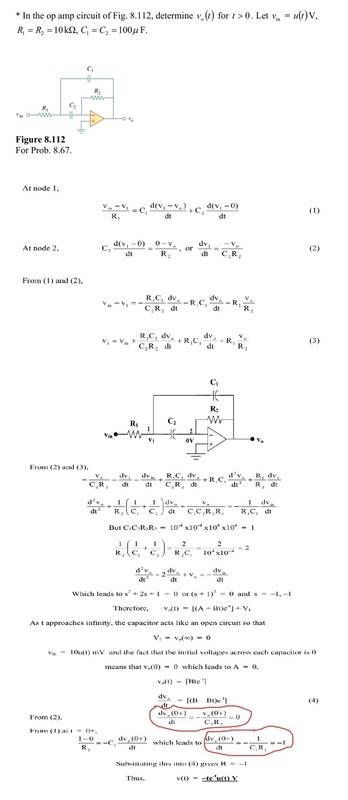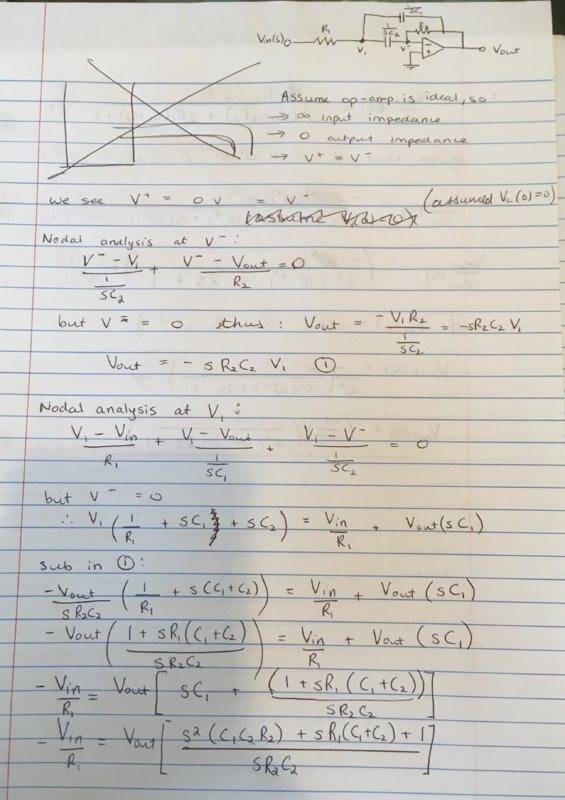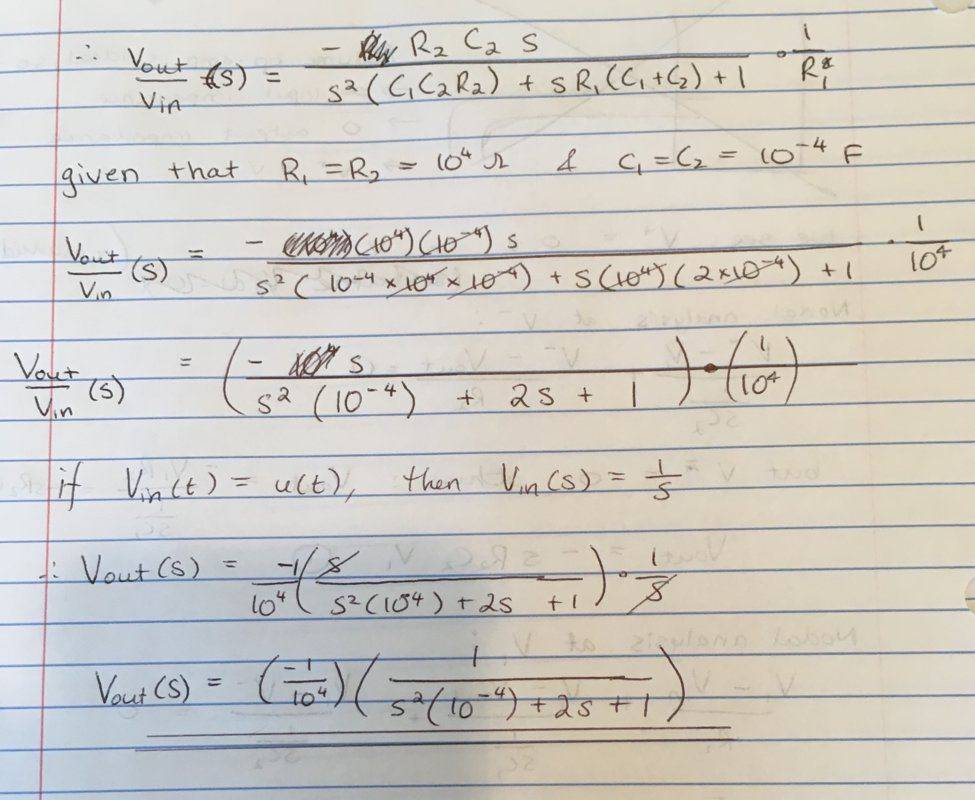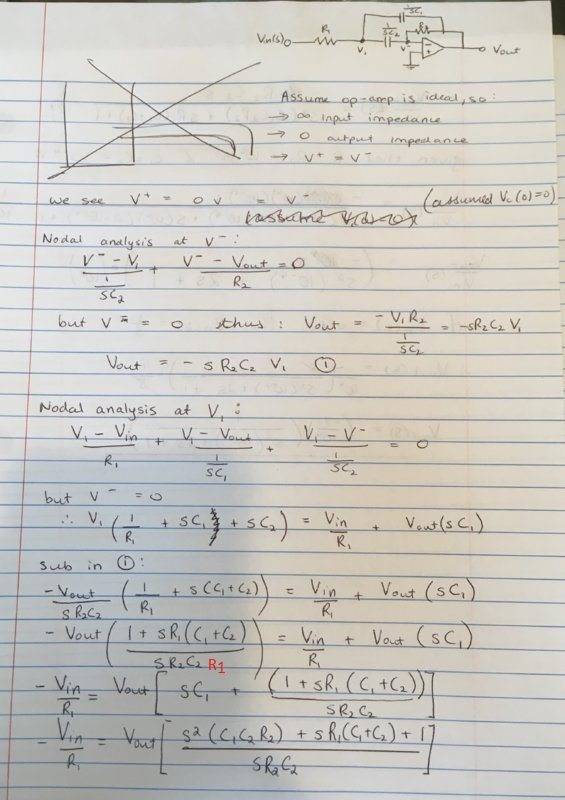# Second order opamp circuit

• Engineering
Homework Statement:
Find the expression for vo(t)
Relevant Equations:
See the attached photo
I was trying to understand the way this problem was solved and I got confused with the latter part of the solution. I encircled the part that confused me. They seem to contradict each other. If dv(0+)/dt = 0 why is it dv(0+)/dt = -1 in the other one? Please explain. TIA!http://i67.tinypic.com/t6s31y.jpg

Last edited:

the scan quality is too poor to be readable.

here's a readable version of the photos

#### Attachments

berkeman
Mentor
here's a readable version of the photos
Thanks for the higher resolution images. I notice that the resolution is fine when I click on the tinypic URL in your first post -- do you have any idea why the image you Uploaded in post #1 is lower resolution?

I also find the equations confusing that you are pointing out. I'll try to spend more time later today looking at it.

I was trying to understand the way this problem was solved and I got confused with the latter part of the solution. I encircled the part that confused me. They seem to contradict each other. If dv(0+)/dt = 0 why is it dv(0+)/dt = -1 in the other one? Please explain. TIA!

Not a direct answer to your question, but have you thought of working through the problem in Laplace space? It saves you from working with all these derivative terms around and should give the same answer.

In direct answer to your question, an initial look leads me to believe that there might be a typo, but I will indeed have a proper look later on.

•gneill and berkeman
I have made an attempt at the problem (apologies for length, but tried to make sure it was clear), here are the images below (I hope that they are legible...). However, when I put my transfer function into MATLAB, I didn't end up with $V_{out}(t) = -t \exp(-t) u(t)$ and I was hoping someone could help point out any errors I made. Thanks.The Electrician
Gold Member
I have made an attempt at the problem (apologies for length, but tried to make sure it was clear), here are the images below (I hope that they are legible...). However, when I put my transfer function into MATLAB, I didn't end up with $V_{out}(t) = -t \exp(-t) u(t)$ and I was hoping someone could help point out any errors I made. Thanks.

You made an algebra mistake; you left out R1 in a denominator. I've shown where it belongs in red. Of course you need to redo all the calculations after that point.•berkeman
You made an algebra mistake; you left out R1 in a denominator. I've shown where it belongs in red. Of course you need to redo all the calculations after that point.

Ah yes, thank you very much. How embarrassing...

For the interest of the OP (@paulmdrdo ), correcting my error yields $$V_{out}(s) = \frac{-1}{s^2 + 2s + 1}$$ taking the inverse Laplace transform of that does indeed yield $V_{out}(t) = -t\exp(-t) u(t)$. I think this way is a much better way of dealing with these problems as you don't have to deal with all these derivative operators around and this allows you to find the response of the circuit to a wide range of inputs, rather than just specifically solving for one type.

Hope that is of some help.

•berkeman
The Electrician
Gold Member
I was trying to understand the way this problem was solved and I got confused with the latter part of the solution. I encircled the part that confused me. They seem to contradict each other. If dv(0+)/dt = 0 why is it dv(0+)/dt = -1 in the other one? Please explain. TIA!
View attachment 241869
http://i67.tinypic.com/t6s31y.jpg
The first thing you have encircled in red says that it's "From (2)". If you go back to (2) you'll see that where the red circled part says first thing: dVo/dt (0+), it should actually be dV1/dt (0+)